# JP6184447B2 - Estimation apparatus and estimation program - Google Patents

## Info

Publication number
JP6184447B2
JP6184447B2 JP2015139792A JP2015139792A JP6184447B2 JP 6184447 B2 JP6184447 B2 JP 6184447B2 JP 2015139792 A JP2015139792 A JP 2015139792A JP 2015139792 A JP2015139792 A JP 2015139792A JP 6184447 B2 JP6184447 B2 JP 6184447B2
Authority
JP
Japan
Prior art keywords
time
integrated value
movement amount
calculated
means
Prior art date
Legal status (The legal status is an assumption and is not a legal conclusion. Google has not performed a legal analysis and makes no representation as to the accuracy of the status listed.)
Active
Application number
JP2015139792A
Other languages
Japanese (ja)
Other versions
JP2017020942A (en
Inventor

Original Assignee

トヨタ自動車株式会社

Priority date (The priority date is an assumption and is not a legal conclusion. Google has not performed a legal analysis and makes no representation as to the accuracy of the date listed.)
Filing date
Publication date
Application filed by 株式会社豊田中央研究所, トヨタ自動車株式会社, 株式会社デンソー, 株式会社Ｓｏｋｅｎ filed Critical 株式会社豊田中央研究所
Priority to JP2015139792A priority Critical patent/JP6184447B2/en
Publication of JP2017020942A publication Critical patent/JP2017020942A/en
Application granted granted Critical
Publication of JP6184447B2 publication Critical patent/JP6184447B2/en
Active legal-status Critical Current
Anticipated expiration legal-status Critical

• 238000004364 calculation methods Methods 0.000 claims description 67
• 238000003384 imaging method Methods 0.000 claims description 56
• 239000011295 pitch Substances 0.000 claims description 46
• 230000000875 corresponding Effects 0.000 claims description 14
• 238000000034 methods Methods 0.000 description 30
• 230000000694 effects Effects 0.000 description 4

## Images

••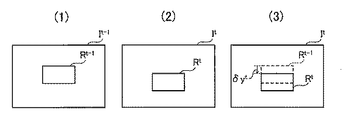•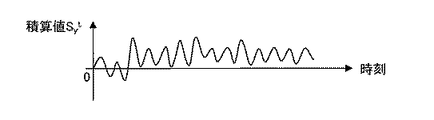•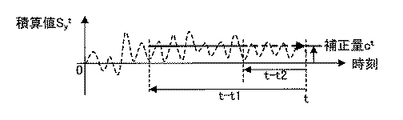•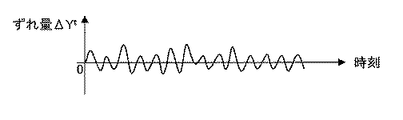•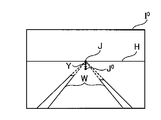•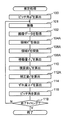•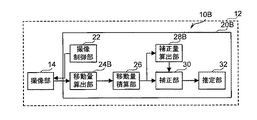•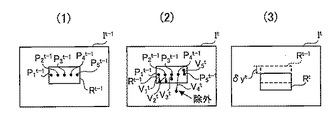•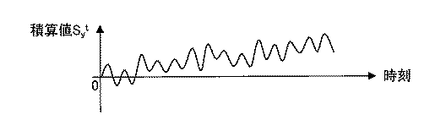•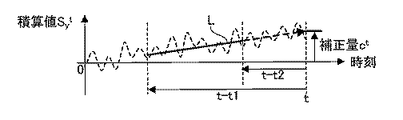•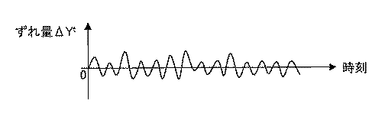•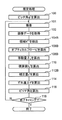## Classifications

• GPHYSICS
• G01MEASURING; TESTING
• G01BMEASURING LENGTH, THICKNESS OR SIMILAR LINEAR DIMENSIONS; MEASURING ANGLES; MEASURING AREAS; MEASURING IRREGULARITIES OF SURFACES OR CONTOURS
• G01B11/00Measuring arrangements characterised by the use of optical means
• G01B11/26Measuring arrangements characterised by the use of optical means for measuring angles or tapers; for testing the alignment of axes
• GPHYSICS
• G06COMPUTING; CALCULATING; COUNTING
• G06TIMAGE DATA PROCESSING OR GENERATION, IN GENERAL
• G06T7/00Image analysis
• G06T7/60Analysis of geometric attributes
• GPHYSICS
• G06COMPUTING; CALCULATING; COUNTING
• G06TIMAGE DATA PROCESSING OR GENERATION, IN GENERAL
• G06T7/00Image analysis
• G06T7/70Determining position or orientation of objects or cameras
• G06T7/73Determining position or orientation of objects or cameras using feature-based methods
• GPHYSICS
• G06COMPUTING; CALCULATING; COUNTING
• G06TIMAGE DATA PROCESSING OR GENERATION, IN GENERAL
• G06T2207/00Indexing scheme for image analysis or image enhancement
• G06T2207/10Image acquisition modality
• G06T2207/10016Video; Image sequence
• GPHYSICS
• G06COMPUTING; CALCULATING; COUNTING
• G06TIMAGE DATA PROCESSING OR GENERATION, IN GENERAL
• G06T2207/00Indexing scheme for image analysis or image enhancement
• G06T2207/30Subject of image; Context of image processing
• G06T2207/30244Camera pose
• GPHYSICS
• G06COMPUTING; CALCULATING; COUNTING
• G06TIMAGE DATA PROCESSING OR GENERATION, IN GENERAL
• G06T2207/00Indexing scheme for image analysis or image enhancement
• G06T2207/30Subject of image; Context of image processing
• G06T2207/30248Vehicle exterior or interior
• GPHYSICS
• G06COMPUTING; CALCULATING; COUNTING
• G06TIMAGE DATA PROCESSING OR GENERATION, IN GENERAL
• G06T2207/00Indexing scheme for image analysis or image enhancement
• G06T2207/30Subject of image; Context of image processing
• G06T2207/30248Vehicle exterior or interior
• G06T2207/30252Vehicle exterior; Vicinity of vehicle

## Description

The present invention relates to an estimation device and an estimation program, and more particularly to an estimation device and an estimation program for estimating a pitch angle of a vehicle.

Conventionally, a technique for estimating the pitch angle of a vehicle based on a plurality of images captured at different times by an imaging unit provided in the vehicle has been proposed. In this technique, based on the plurality of images, a translation vector indicating a moving direction of the imaging unit and a rotation matrix indicating an angle change amount in the pitch direction of the posture of the imaging unit are calculated.

In this technique, the first pitch angle estimated value is sequentially calculated based on the direction of the translation vector, and the second pitch angle estimated value is calculated by integrating the angle change amount indicated by the rotation matrix. In this technique, the pitch angle of the vehicle is corrected by correcting the second pitch angle estimated value in accordance with the deviation between the average value of the first pitch angle estimated value and the average value of the second pitch angle estimated value in a predetermined period. Determine an estimate of.

JP 2012-026992 A

In the technique of Patent Document 1, the pitch angle of the vehicle is estimated based on the restriction that the translation vector is parallel to the road surface. Therefore, in the technique disclosed in Patent Document 1, when the translation vector is relatively small, that is, when the vehicle speed is relatively low, the pitch angle of the vehicle cannot be estimated with high accuracy.

The present invention has been made in view of the above circumstances, and provides an estimation device and an estimation program capable of accurately estimating the pitch angle of a vehicle even when the vehicle speed is relatively low. With the goal.

In order to achieve the above object, an estimation apparatus according to the present invention is provided in a vehicle and is imaged by an imaging unit that captures at least one of the front and rear images of the vehicle and the imaging unit at a first time. The predetermined area based on the position of the predetermined area in the image and the position of the area corresponding to the predetermined area in the image captured in the same direction by the imaging means at the second time after the first time. A calculating means for calculating the amount of movement in the vertical direction of the region; an integrating means for calculating an integrated value of the moving amount each time the moving amount is calculated by the calculating means; and the integrating means within a past predetermined period. Based on the time-series data of the integrated value of the movement amount calculated by the correction unit, the correcting unit correcting the present integrated value calculated by the integrating unit, and the integrated value corrected by the correcting unit. Te, including an estimation means for estimating a pitch angle of the vehicle.

The estimation program according to the present invention includes a computer provided in a vehicle, and a position of a predetermined region in an image captured at a first time by an imaging unit that captures at least one of the front and rear images of the vehicle, and A vertical movement amount of the predetermined area is calculated based on a position of an area corresponding to the predetermined area in an image captured in the same direction by the imaging unit at a second time after the first time. A calculating unit; an integrating unit that calculates an integrated value of the moving amount each time the moving amount is calculated by the calculating unit; and an integrated value of the moving amount calculated by the integrating unit within a predetermined past period. Correction means for correcting the current integrated value calculated by the integrating means based on the time series data, and the vehicle pitch based on the integrated value corrected by the correcting means. And estimating means for estimating a corner is a program for functioning as a.

According to the present invention, the position of the predetermined region in the image captured at the first time by the imaging unit that is provided in the vehicle by the calculation unit and captures at least one of the front and rear images of the vehicle, and the first Based on the position of the area corresponding to the predetermined area in the image captured in the same direction by the imaging means at a second time after one time, the vertical movement amount of the predetermined area is calculated. .

Further, each time the moving amount is calculated by the calculating unit, an integrated value of the moving amount is calculated by the integrating unit. Then, the current integrated value calculated by the integrating means is corrected by the correcting means based on the time-series data of the integrated value of the movement amount calculated by the integrating means in the past predetermined period. Further, the pitch angle of the vehicle is estimated by the estimation unit based on the integrated value corrected by the correction unit.

Thus, according to the present invention, since the current integrated value is corrected based on the time-series data of the integrated value within a predetermined period in the past, even when the vehicle speed is relatively low. The pitch angle of the vehicle can be accurately estimated.

In the estimation apparatus of the present invention, the correction unit may correct the current integrated value using an average value of time-series data of the integrated value of the movement amount as a correction amount.

In the estimation apparatus of the present invention, the correction means calculates an extrapolated value obtained by approximating the time series data of the integrated value of the movement amount as a correction amount, and uses the correction amount to calculate the current integrated value. May be corrected.

In the estimation apparatus of the present invention, the correction unit is configured to calculate the image from the steady state at the current time based on the time-series data of the integrated value of the movement amount and the current integrated value calculated by the integration unit. As the amount of deviation in the vertical direction, the corrected integrated value at the present time may be calculated.

In the estimation apparatus of the present invention, the predetermined area may be an area including a vanishing point in perspective.

In the estimation apparatus of the present invention, the correction unit may correct the current integrated value by setting the predetermined period to be longer as the correction time becomes later.

In the estimation apparatus of the present invention, the calculation unit may calculate the movement amount after searching for the predetermined area at the second time corresponding to the predetermined area at the first time by pattern matching.

The storage medium for storing the estimation program according to the present invention is not particularly limited, and may be a hard disk or a ROM. Further, it may be a CD-ROM, a DVD disk, a magneto-optical disk or an IC card. Furthermore, the estimation program may be downloaded from a server or the like connected to the network.

According to the present invention, there is an effect that the pitch angle of the vehicle can be accurately estimated even when the vehicle speed is relatively low.

It is a block diagram which shows the structure of the estimation apparatus which concerns on 1st Embodiment. It is the schematic where it uses for description of the moving amount | distance in the perpendicular direction of the predetermined area | region in the image obtained by the imaging by the imaging part which concerns on 1st Embodiment. It is a graph which shows the time series data of the integrated value of the moving amount | distance which concerns on 1st Embodiment. It is a graph with which it uses for description of the calculation process of the corrected amount which concerns on 1st Embodiment. It is a graph which shows the time series data of the deviation | shift amount of the perpendicular direction which concerns on 1st Embodiment. It is the schematic where it uses for description of the calculation process of the deviation | shift amount of the perpendicular direction in the steady state which concerns on each embodiment. It is a flowchart which shows the flow of the estimation process which concerns on 1st Embodiment. It is a block diagram which shows the structure of the estimation apparatus which concerns on 2nd Embodiment. It is the schematic where it uses for description of the calculation process of the optical flow which concerns on 2nd Embodiment. It is a graph which shows the time series data of the integrated value of the moving amount | distance which concerns on 2nd Embodiment. It is a graph with which it uses for description of the calculation process of the corrected amount which concerns on 2nd Embodiment. It is a graph which shows the time series data of the deviation | shift amount of the perpendicular direction which concerns on 2nd Embodiment. It is a flowchart which shows the flow of the estimation process which concerns on 2nd Embodiment.

Embodiments for carrying out the present invention will be described below in detail with reference to the drawings.

[First Embodiment]
First, the configuration of estimation apparatus 10A according to the present embodiment will be described with reference to FIG.

As illustrated in FIG. 1, the estimation device 10A according to the present embodiment includes an imaging unit 14 and a computer 20A that are provided in the vehicle 12, respectively.

The imaging unit 14 according to the present embodiment is, for example, a monocular in-vehicle camera, and controls images from the front of the vehicle 12 at predetermined time intervals (33 milliseconds as an example in the present embodiment) under the control of the computer 20A. And sequentially output image data indicating the captured image to the computer 20A. Note that the imaging unit 14 may capture a color image, or may capture a monochrome image. In addition, the imaging unit 14 may be capable of capturing moving images, or may be capable of capturing still images in continuous shooting.

The computer 20A according to the present embodiment includes a CPU, a RAM, a ROM that stores an estimation processing program, which will be described later, and a nonvolatile storage unit, and is functionally configured as described below. The computer 20A includes an imaging control unit 22, a movement amount calculation unit 24A, a movement amount integration unit 26, a correction amount calculation unit 28A, a correction unit 30, and an estimation unit 32. The imaging unit 14 is an example of an imaging unit, and the movement amount calculation unit 24A is an example of a calculation unit. The movement amount integrating unit 26 is an example of an integrating unit, the correction amount calculating unit 28A and the correcting unit 30 are examples of a correcting unit, and the estimating unit 32 is an example of an estimating unit.

The imaging control unit 22 according to the present embodiment controls the imaging unit 14 to capture images in front of the vehicle 12 at the predetermined time intervals.

The movement amount calculation unit 24A according to the present embodiment sequentially acquires the image data output by the imaging unit 14 at predetermined time intervals. Then, the movement amount calculation unit 24A uses the image data acquired at the current time t and the image data acquired at the time t-1 that is one time after the predetermined period interval from the current time, to be described later. t is calculated. Hereinafter, the process of calculating the movement amount δy t by the movement amount calculation unit 24A will be described in detail with reference to FIG.

FIG. 2 (1) shows an image It -1 shown by the image data acquired at time t-1 by the movement amount calculation unit 24A. FIG. 2 (2) shows the image It -1 at time t by the movement amount calculation unit 24A. It shows an image I t indicated by the acquired image data. Also, FIG. 2 (3) shows the movement amount .delta.y t in the vertical direction (pitching direction of the vehicle 12) to the area R t-1 region R t in the image I t.

First, as shown in FIG. 2 (1), the movement amount calculating section 24A, from the image data representing the image I t-1, the predetermined including the predetermined position in the image I t-1 the size of the rectangular area Image data indicating an image in R t-1 is extracted. Specifically, the movement amount calculation unit 24A according to the present embodiment, for example, is a third of the entire imaging region in both the vertical direction and the horizontal direction in FIG. 2 (1) including the center of the entire imaging region. Image data indicating an image in a rectangular region R t- 1 having a length of 1 is extracted.

Next, as shown in FIG. 2 (2), the movement amount calculating section 24A is by pattern matching, searching for a region R t corresponding to the region R t-1 in the image I t. Specifically, the shift amount calculating part 24A according to this embodiment, as an example, from the position of the upper left point of the image I t 2 (2), of the same shape and size as the region R t-1 region The image data indicating the image in the region, the image data indicating the image in the region R t−1 , and the right position are shifted to the right end by a predetermined number of pixels (3 pixels in the present embodiment) by a predetermined number of pixels. The degree of the correlation is calculated. Further, the shift amount calculating part 24A is the process of the calculation, a predetermined number of pixels (in this embodiment, two pixels) in the downward direction in each Fig. 2 (2) is repeated until the lower end of the image I t.

The movement amount calculating section 24A determines a region R t the highest region degree indicating the correlation. In the present embodiment, as the region having the highest degree of correlation, a region where the sum of all the pixels of the absolute value in the difference between the pixel values of the corresponding pixels is minimized is applied. It is not limited to this. For example, as the region having the highest degree of correlation, the sum of all pixels of the absolute value in the pixel value difference between pixels at predetermined positions for each of a plurality of corresponding pixel groups, such as 2 pixels × 2 pixels. A region that minimizes may be applied.

Then, as shown in FIG. 2 (3), the movement amount calculation unit 24A sets the movement amount δy t as the movement amount δy t at the corresponding position between the region R t-1 and the region R t (in FIG. 2 (3), The number of pixels in the vertical direction (vertical direction in FIG. 2 (3)) between the pixel positions indicating (position) is calculated.

Thus, the movement amount calculating section 24A according to this embodiment, although the region in the degree highest image I t representing the correlation determines the region R t, are not limited thereto. For example, the movement amount calculating section 24A, of the area in the image I t, one of the region above the threshold degree indicating the correlation is predetermined may be determined as a region R t.

In this case, for example, the movement amount calculation unit 24A starts the search and first determines a region where the degree of the correlation is equal to or greater than the threshold value as the region Rt . Thus, although search accuracy region R t by the movement amount calculating section 24A is likely to drop, since better even without the processing of the search for all the range of the image I t by the movement amount calculating section 24A The amount of calculation by the movement amount calculation unit 24A is reduced.

The movement amount integrating unit 26 according to the present embodiment uses the following equation (1) to calculate the movement amount sequentially calculated by the movement amount calculating unit 24A as the integrated value S y t of the movement amount δy t at time t. An integrated value of δy t is calculated.

FIG. 3 shows an example of time-series data of the integrated value S y t calculated by the movement amount integrating unit 26. The vertical axis of FIG. 3 shows the integrated value S y t, the horizontal axis represents time. As shown in FIG. 3, the integrated value S y t becomes an offset value as time passes.

As described above, this is because the movement amount accumulation unit 26 accumulates the movement amount δy t sequentially calculated by the movement amount calculation unit 24A, so that errors included in the movement amount δy t are also accumulated. It is. Note that this error is, for example, described above by the movement amount calculating section 24A region R t influence of the image processing for exploring, and the region R t-1 within the time imaged object itself from time t-1, t This is due to the effects of moving during the period.

Therefore, the correction amount calculation unit 28A according to the present embodiment performs the above error based on the time-series data of the integrated value S y t of the movement amount δy t calculated by the movement amount integration unit 26 in the past predetermined period. It calculates a correction amount c t for correcting. Referring to FIG. 4, it will be described in detail calculation of the correction amount c t by the correction amount calculation unit 28A. Incidentally, in FIG. 4, the time-series data of integrated value S y t shown in FIG. 3 are indicated by broken lines. Similarly to FIG. 3, the vertical axis of FIG. 4 indicates the integrated value S y t , and the horizontal axis indicates the time.

As shown in FIG. 4, the correction amount calculation unit 28A according to the present embodiment uses the following equation (2) as a correction amount ct at time t as a past predetermined period (time t− in FIG. 4). An average value of the integrated values S y t calculated at each time of the predetermined period interval (period from t1 to time t−t2) is calculated.

However, T1 indicates the number of times that the integrated value S y t has been calculated from time t-t1 to time t, and T2 indicates the number of times that the integrated value S y t has been calculated from time t-t2 to time t. Show.

In the present embodiment, the pitch angle estimation accuracy of the vehicle 12, which will be described later, is within an allowable range as a result of the past predetermined period, through experiments using actual machines of the vehicle 12, computer simulations based on the design specifications of the vehicle 12, or the like. Although the inner period is fixedly applied, the present invention is not limited to this. As described above, since the error is also integrated, a longer period may be applied as the predetermined period as the time point at which the correction amount ct is calculated is later.

Correcting unit 30 according to the present embodiment, by using the correction amount c t calculated by the correction amount calculation unit 28A, it corrects the integrated value S y t calculated by the movement amount integrating section 26. Specifically, the correction unit 30 subtracts the correction amount c t from the integrated value S y t by using the following equation (3), whereby the current position with respect to the vertical position of the horizontal line in the image in the steady state is determined. The amount of deviation ΔY t in the vertical position of the horizontal line in the image at time t is calculated. Note that, as the steady state here, for example, the vehicle 12 is substantially swayed in the vertical direction, such as a state where the vehicle 12 is stopped or a state where the movement amount δy t ≈0 described above continues for a long period of time. There is no state.

FIG. 5 shows time series data of the deviation amount ΔY t obtained by correcting the time series data of the integrated value S y t shown in FIG. 3 by the correction unit 30 at each time for each predetermined period interval. Has been. Incidentally, the vertical axis is the shift amount [Delta] Y t in FIG. 5, the horizontal axis represents time.

It will now be described in detail deviation amount [Delta] Y t. The position Y t in the vertical direction of the horizontal line in the image captured by the imaging unit 14 at time t is expressed by the following equation (4).
However, Y 0 denotes the vertical position of a horizontal line in the steady state.

In general, the position Y t at the time t fluctuates around the position Y 0 in the steady state. Therefore , the average value of the deviation amounts ΔY t calculated at each time up to the time t is almost 0 ( Equal to zero). Further, the integrated value S y t, if not included the error in the amount of movement .delta.y t is is equal to the deviation amount △ Y t. In other words, in this case, the average value of the integrated values S y t is also substantially equal to 0 (zero).

However, as described above, the integrated value S y t includes the error of the movement amount δy t . Therefore, in this embodiment, as described above, as the correction amount c t used for correction of the error, the mean value of the integrated value S y t calculated for each time point for each said predetermined period intervals of the predetermined time period Calculated.

The estimation unit 32 according to the present embodiment estimates the pitch angle of the vehicle 12 based on the integrated value S y t (= shift amount ΔY t ) corrected by the correction unit 30. Specifically, the estimation unit 32 calculates the pitch angle φ t of the imaging unit 14 from the shift amount ΔY t using the specification values such as the resolution and the focal length of the imaging unit 14.

Since the pitch angle φ t is the angle of the optical axis of the imaging unit 14 at time t with respect to the optical axis of the imaging unit 14 in the steady state, the estimation unit 32 is calculating a pitch angle phi 0 of the optical axis. And the estimation part 32 calculates the angle which added pitch angle (phi) 0 which is an angle with respect to the road surface of the optical axis of the imaging part 14, and pitch angle (phi) t as a pitch angle of the vehicle 12 in the time t.

Here, with reference to FIG. 6, the calculation process of the pitch angle φ 0 in the steady state will be described. Here, in order to avoid complications, as an example, it is assumed that the imaging unit 14 is an imaging unit in which the optical axis is positioned at the center of the image when the pitch angle of the optical axis with respect to the road surface is 0 degrees. FIG. 6 shows an example of an image I 0 captured by the imaging unit 14 in the steady state.

As shown in FIG. 6, the estimation unit 32 regards the intersection J of the two white lines W as the vertical position of the horizontal line H, and uses the intersection J and the vehicle as the amount of displacement Y of the horizontal line position in the steady state. The number of pixels in the vertical direction (vertical direction in FIG. 6) between the pixel positions of the image I 0 and the intersection J 0 obtained in advance for the case where the pitch angle is 0 degree is calculated. Then, the estimation unit 32 calculates the pitch angle φ 0 from the deviation amount Y using specification values such as the resolution and focal length of the imaging unit 14. Note that the calculation processing of the pitch angle φ 0 in the steady state is not limited to this, and other known methods may be used.

Next, with reference to FIG. 7, the operation of estimation apparatus 10A according to the present embodiment will be described. FIG. 7 is a flowchart showing the flow of processing of an estimation processing program executed by the CPU of the computer 20A when, for example, the ignition switch of the vehicle 12 is turned on.

In step 100 of FIG. 7, the estimation unit 32 controls the imaging unit 14 to capture an image ahead of the vehicle 12 as described above, and the pitch angle in a steady state from the image I 0 obtained by the imaging. to calculate the φ 0.

In the next step 101, the imaging control unit 22 controls the imaging unit 14 to capture an image in front of the vehicle 12. In the next step 102, the movement amount calculating section 24A acquires the image data representing the image I t output from the imaging unit 14 by the processing of step 101.

In the next step 104A, the movement amount calculation unit 24A, from the image data acquired by the previous processing of step 102 in the repetitive processing of step 118 to step 118 described later, the image I t− indicated by the image data. extracts image data indicating an image of one region R t-1. In addition, the process of this step 104A and the process of step 106A-step 116 mentioned later are not performed at the timing performed for the first time after starting execution of this estimation process program.

In the next step 106A, the shift amount calculating part 24A, as described above, the area extracted by the processing of step 104A in the image I t indicated by the image data obtained by the preceding process in step 102 R t A region R t corresponding to −1 is searched.

In the next step 108A, the shift amount calculating part 24A, as described above, the region R t-1 extracted by the processing of step 104A, corresponding to the searched region R t by the processing of step 106A The movement amount δy t is calculated based on the pixel position.

In the next step 110, as described above, the movement amount integrating unit 26 calculates the integrated value S y t of the movement amount δy t calculated by the processing of step 108A using the above equation (1).

In the next step 112A, the correction amount calculation unit 28A uses the above expression (2), as the correction amount c t, the average of the past accumulated value calculated by the process at step 110 in a predetermined time period S y t Calculate the value.

In the next step 114, the correction unit 30 subtracts the correction amount c t calculated by the process of step 112A from the integrated value S y t calculated by the process of step 110, using the equation (3). As a result, a deviation amount ΔY t is calculated.

In the next step 116, the estimation unit 32 calculates the pitch angle φ t from the deviation amount ΔY t calculated by the processing in step 114, using the specification values of the imaging unit 14 as described above. Then, as described above, the estimation unit 32 calculates the pitch angle of the vehicle 12 by adding the pitch angle φ t and the pitch angle φ 0 calculated by the processing of step 100. Note that the pitch angle calculated here is, for example, 2 when estimating the distance to an object in an image in front of the vehicle 12 imaged by imaging means such as a camera, for example, with advanced driving assistance of the vehicle 12. This is used when three-dimensional information is estimated from dimensional information.

In step 118, the estimation unit 32 determines whether or not a predetermined end timing has come. If this determination is negative, the process returns to step 101. If the determination is affirmative, this estimation processing program is terminated. In the present embodiment, as the predetermined end timing, for example, a timing at which the ignition switch of the vehicle 12 is turned off is applied.

As described above, for example, when the movement amount δy t calculated by the process of step 108A continues to be approximately 0 (zero) for a long time (that is, the steady state), the integrated value S y t May be reset to 0 (zero), and the estimation processing program may be executed again from the processing of step 100.

As described above, according to the present embodiment, the current integrated value S y t is corrected based on the time-series data of the integrated value S y t within a predetermined period in the past. Even when the speed is relatively low, the pitch angle of the vehicle 12 can be accurately estimated using the monocular imaging unit 14. Further, according to the present embodiment, it is possible to accurately estimate the pitch angle of the vehicle 12 with a small amount of calculation compared to the case where the translation vector and the rotation matrix are calculated.

[Second Embodiment]
First, the configuration of estimation apparatus 10B according to the present embodiment will be described with reference to FIG. In FIG. 8, components having the same functions as those in FIG. 1 are denoted by the same reference numerals as those in FIG. 1, and description thereof is omitted.

As shown in FIG. 8, the estimation apparatus 10B according to the present embodiment is different from the estimation apparatus 10A according to the first embodiment in that a computer 20B is provided instead of the computer 20A. Further, the computer 20B according to the present embodiment includes a movement amount calculation unit 24B instead of the movement amount calculation unit 24A, and a correction amount calculation unit 28B instead of the correction amount calculation unit 28A. Is different from the computer 20A according to the first embodiment.

The movement amount calculation unit 24B according to the present embodiment sequentially acquires the image data output by the imaging unit 14 at predetermined time intervals. Then, the movement amount δy t is calculated by using the image data acquired at the current time t and the image data acquired at the time t−1 that is one predetermined time interval backward from the current time. Hereinafter, with reference to FIG. 9, the process of calculating the movement amount δy t by the movement amount calculation unit 24B will be described in detail.

9 (1), the point used for calculating the image I t-1, and described below optical flow V i t indicated by the image data acquired at time t-1 by the movement amount calculating section 24B (hereinafter, "calculation It is referred to as “point”.) P i t−1 ((i = 1... N (N is a natural number. In the present embodiment, 5))). Further, FIG. 9 (2) illustrates an image I t, and later to the optical flow V i t indicated by the image data acquired at time t by the movement amount calculating section 24B. Further, FIG. 9 (3) shows the movement amount δy t as in FIG. 2 (3).

First, as shown in FIG. 9 (1), the movement amount calculating section 24B, from the image data representing the image I t-1, the predetermined in an image I t-1 the size of the rectangular area R t-1 Image data representing the image inside is extracted. In the present embodiment, a region including a vanishing point in perspective is applied as the region R t-1 when the vehicle 12 travels in a state where no pitching of the vehicle 12 occurs. This is because the region is a region that can be regarded as a region in which movement due to the influence of the traveling of the vehicle 12 is relatively small.

Next, as illustrated in FIG. 9A, the movement amount calculation unit 24B determines N predetermined positions in the region R t−1 as the positions of the calculation points P i t−1 . In the present embodiment, as an example, the position of the calculation point P i t-1 is the center position of the region R t-1 with respect to the vertical direction (vertical direction in FIG. 9 (1)), and is horizontal. For the direction (the horizontal direction in FIG. 9 (1)), the positions spaced apart from each other are applied. Thus, in this embodiment, as the position of the calculation point P i t-1, was the application of the previously fixedly defined position, but is not limited thereto. For example, as the position of the calculation point P i t-1, Harris operator ( "C.Harris and M.Stephens (1988)." A combined corner and edge detector "." Proceedings of the 4th Alvey Vision Conference ". Pp.147 -151 ")) or the like, a position where a corresponding point between a plurality of images can be easily matched may be applied.

Also, as shown in FIG. 9 (2), the movement amount calculation unit 24B performs an optical flow V i t (= {vx i t , vy i t }) at time t for each calculation point P i t−1. Calculate each. Here, the vx i t represents the x component of the optical flow V i t (components in the horizontal direction), the vy i t represents the y component of the optical flow V i t (components in the vertical direction). In the present embodiment, as a method used for calculating the optical flow V i t, was the application of the block matching method is not limited thereto. For example, other techniques such as the lucas-kanade method may be applied as the technique.

Further, the movement amount calculation unit 24B calculates the average value of the y component (vy i t ) of the optical flow V i t as the movement amount δy t . The movement amount calculating section 24B according to this embodiment, the when calculating the average value, a predetermined range as the range value of the y component of the optical flow V i t is normal value Optical The flow V i t (V 4 t shown in FIG. 9 (2)) is not counted. Specifically, for example, the movement amount calculating section 24B, when calculating the average value, the difference between the average value of the y component of another optical flow V i t is a predetermined range (for example, the Optical flows V i t that are outside the range of ± 50% of the average value are not counted.

As described above, in this embodiment, the average value of the y component values of the optical flow V i t is applied as the movement amount δy t , but is not limited to this. For example, the median value of the y component values of the optical flow V i t may be applied as the movement amount δy t .

The correction amount calculation unit 28B according to the present embodiment uses the correction amount c t based on the time series data of the integrated value S y t of the movement amount δy t calculated by the movement amount integration unit 26 in the past predetermined period. Is calculated. Hereinafter, with reference to FIGS. 10 and 11, it will be described in detail calculation of the correction amount c t by the correction amount calculation unit 28B. Note that FIG. 10 shows time-series data of the integrated value S y t as in FIG. 3. Further, in FIG. 11, similar to FIG. 4, the time-series data of integrated value S y t shown in FIG. 10 is shown in broken lines.

The error included in the movement amount δy t described in the first embodiment is not necessarily a constant value. Therefore, in the present embodiment, as shown in FIG. 11, the correction amount calculation unit 28 </ b> B performs the past predetermined period (period from time t-t1 to time t-t2 in FIG. 11) for each predetermined period. By approximating the integrated value S y t calculated at each time using, for example, the least square method or the like, a linear coefficient and a constant indicating a linear expression representing the regression line L are calculated. Then, the correction amount calculating section 28B is by extrapolation using the linear expression to calculate the correction amount c t at time t. Incidentally, in FIG. 12, like FIG. 5, the time-series data of integrated value S y t shown in FIG. 11, when the shift amount [Delta] Y t corrected by the correction unit 30 at each time for each said predetermined period intervals Series data is shown.

As described above, in the present embodiment, the time series data of the integrated value S y t is approximated to a linear expression, but the present invention is not limited to this. For example, time-series data of integrated value S y t may be approximated to the quadratic equation, etc., by extrapolation the correction amount c t integrated value S y time t from the time series data of t in the past predetermined period As long as calculation is possible, the approximation method is not limited.

Next, with reference to FIG. 13, the effect | action of the estimation apparatus 10B which concerns on this Embodiment is demonstrated. FIG. 13 is a flowchart showing the flow of processing of the estimation processing program executed by the CPU of the computer 20B when the ignition switch of the vehicle 12 is turned on, for example. Further, steps in FIG. 13 that execute the same processing as in FIG. 7 are assigned the same step numbers as in FIG.

In step 104B of FIG. 13, the movement amount calculation unit 24B determines from the image data acquired by the previous processing of step 102 in the above repetitive processing in the region R t-1 of the image It -1 indicated by the image data. The image data indicating the image is extracted.

In the next step 106B, as described above, the movement amount calculation unit 24B calculates the N predetermined positions in the region R t-1 indicated by the image data extracted by the process in step 104B. The position of the point P i t-1 is determined. Then, as described above, the movement amount calculation unit 24B uses the image data extracted by the process of step 104B and the image data acquired by the process of step 102 just before, to calculate each calculation point P i t. for -1, calculates the optical flow V i t.

In the next step 108B, as described above, the movement amount calculation unit 24B sets the y component of the optical flow V i t at each calculation point P i t−1 calculated by the processing in step 106B as the movement amount δy t. The average value of is calculated.

Thereafter, in step 112B, as described above, the correction amount calculation unit 28B is based on the time-series data of the integrated value S y t of the movement amount δy t calculated by the movement amount calculation unit 24B in the past predetermined period. The correction amount ct is calculated.

As described above, according to the present embodiment, the same effects as those of the first embodiment can be obtained.

In the first embodiment, a region including the vanishing point may be applied as the region R t-1 as in the second embodiment. Further, in the above first embodiment, as hereinabove described second embodiment, may be calculated movement amount .delta.y t using optical flow V i t. Further, in the above first embodiment, as hereinabove described second embodiment, by approximating the time-series data of integrated value S y t within a past predetermined period, the correction amount c t by extrapolation It may be calculated. Also in the second embodiment, if the movement amount δy t continues to be approximately 0 (zero) for a long time, the integrated value S y t is reset to 0 (zero) and the step is performed again. The estimation processing program may be executed again from 100 processes.

Moreover, although each said embodiment demonstrated the case where the image ahead part of the vehicle 12 was imaged with the imaging part 14, this invention is not limited to this. For example, the image capturing unit 14 may capture an image behind the vehicle 12. In this case, for example, the pitch angle of the vehicle 12 is calculated as in the above embodiments, and the angle obtained by reversing the sign of the pitch angle is estimated as the pitch angle of the vehicle 12.

Moreover, you may combine said each embodiment and the said form example which images the image of the back of the vehicle 12 by the imaging part 14. FIG. In this case, as the absolute value of the correction amount c t for correcting the error is small, is considered to said error is small. Therefore, using this case, for example, of the correction amount c t calculated using each of an image obtained by each imaging the front and rear of the vehicle 12, the correction amount c t towards absolute value is smaller Thus, a mode of calculating the pitch angle of the vehicle 12 is exemplified. In addition, for example, as the pitch angle of the vehicle 12, a mode of calculating the average value of the pitch angles calculated using each of the images obtained by imaging the front and the rear of the vehicle 12 is also exemplified.

In each of the above embodiments, the case where a rectangle is applied as the shape of the region R has been described. However, the present invention is not limited to this. For example, it is needless to say that other shapes such as a circle and a triangle may be used as long as the movement amount δy t can be calculated from an image obtained by imaging by the imaging unit 14.

10A, 10B estimation device 12 vehicle 14 imaging unit 20A, 20B computer 22 imaging control unit 24A, 24B movement amount calculation unit 26 movement amount integration unit 28A, 28B correction amount calculation unit 30 correction unit 32 estimation unit

## Claims (8)

1. An imaging means provided in the vehicle for capturing at least one of the front and rear images of the vehicle;
The position of the predetermined area in the image captured by the imaging means at the first time and the predetermined area in the image captured in the same direction by the imaging means at the second time after the first time. Calculation means for calculating a vertical movement amount of the predetermined area based on the position of the corresponding area;
An integration unit that calculates an integrated value of the movement amount each time the movement amount is calculated by the calculation unit;
Correction means for correcting the current integrated value calculated by the integrating means based on time-series data of the integrated value of the movement amount calculated by the integrating means in a past predetermined period;
Estimating means for estimating a pitch angle of the vehicle based on the integrated value corrected by the correcting means;
Including the estimation device.
2. The estimation device according to claim 1, wherein the correction unit corrects the current integrated value using an average value of time-series data of the integrated value of the movement amount as a correction amount.
3. The correction means calculates an extrapolated value obtained by approximating time series data of the integrated value of the movement amount as a correction amount, and corrects the current integrated value using the correction amount. Estimating device.
4. The correction means, based on the time series data of the integrated value of the movement amount and the current integrated value calculated by the integrating means, as the vertical deviation amount of the image from the steady state at the current time, The estimation apparatus according to any one of claims 1 to 3, wherein the corrected integrated value at the present time is calculated.
5. The estimation device according to claim 1, wherein the predetermined region is a region including a vanishing point in perspective.
6. The estimation device according to any one of claims 1 to 5, wherein the correction unit corrects the current integrated value by setting the predetermined period to be a longer period as the correction time becomes later.
7. The calculation unit calculates the movement amount after searching the predetermined area at the second time corresponding to the predetermined area at the first time by pattern matching. The estimation device described.
8. Computer
A position of a predetermined region in an image captured at a first time by an imaging means provided on the vehicle and capturing at least one of the front and rear images of the vehicle; and the second time after the first time Calculation means for calculating a vertical movement amount of the predetermined area based on a position of an area corresponding to the predetermined area in an image imaged in the same direction by the imaging means;
An integration unit that calculates an integrated value of the movement amount each time the movement amount is calculated by the calculation unit;
Correction means for correcting the current integrated value calculated by the integrating means based on time-series data of the integrated value of the movement amount calculated by the integrating means in a past predetermined period;
Estimating means for estimating a pitch angle of the vehicle based on the integrated value corrected by the correcting means;
Estimating program to function as.
JP2015139792A 2015-07-13 2015-07-13 Estimation apparatus and estimation program Active JP6184447B2 (en)

## Priority Applications (1)

Application Number Priority Date Filing Date Title
JP2015139792A JP6184447B2 (en) 2015-07-13 2015-07-13 Estimation apparatus and estimation program

## Applications Claiming Priority (3)

Application Number Priority Date Filing Date Title
JP2015139792A JP6184447B2 (en) 2015-07-13 2015-07-13 Estimation apparatus and estimation program
US15/735,357 US10422634B2 (en) 2015-07-13 2016-06-24 Estimating device and estimating program
PCT/JP2016/068920 WO2017010268A1 (en) 2015-07-13 2016-06-24 Estimation device and estimation program

## Publications (2)

Publication Number Publication Date
JP2017020942A JP2017020942A (en) 2017-01-26
JP6184447B2 true JP6184447B2 (en) 2017-08-23

# Family

## Family Applications (1)

Application Number Title Priority Date Filing Date
JP2015139792A Active JP6184447B2 (en) 2015-07-13 2015-07-13 Estimation apparatus and estimation program

## Country Status (3)

US (1) US10422634B2 (en)
JP (1) JP6184447B2 (en)
WO (1) WO2017010268A1 (en)

## Families Citing this family (1)

* Cited by examiner, † Cited by third party
Publication number Priority date Publication date Assignee Title
WO2019216393A1 (en) * 2018-05-10 2019-11-14 パナソニックＩｐマネジメント株式会社 Road surface state estimating method, road surface state estimating device, and road surface state estimating system

## Family Cites Families (8)

* Cited by examiner, † Cited by third party
Publication number Priority date Publication date Assignee Title
US5638116A (en) * 1993-09-08 1997-06-10 Sumitomo Electric Industries, Ltd. Object recognition apparatus and method
JP4586571B2 (en) 2005-02-25 2010-11-24 株式会社豊田中央研究所 Object judgment device
JP2006012191A (en) * 2005-09-01 2006-01-12 Honda Motor Co Ltd Lane marking recognition device for vehicle
US8164628B2 (en) * 2006-01-04 2012-04-24 Mobileye Technologies Ltd. Estimating distance to an object using a sequence of images recorded by a monocular camera
EP3112802B1 (en) * 2007-02-16 2019-10-09 Mitsubishi Electric Corporation Road feature measurement apparatus and road feature measuring method
JP5570902B2 (en) * 2010-07-28 2014-08-13 本田技研工業株式会社 Vehicle pitch angle estimation device
JP6011489B2 (en) * 2013-08-26 2016-10-19 トヨタ自動車株式会社 In-vehicle control device
CN107438754A (en) * 2015-02-10 2017-12-05 御眼视觉技术有限公司 Sparse map for autonomous vehicle navigation

## Also Published As

Publication number Publication date
JP2017020942A (en) 2017-01-26
WO2017010268A1 (en) 2017-01-19
US20180180409A1 (en) 2018-06-28
US10422634B2 (en) 2019-09-24

## Similar Documents

Publication Publication Date Title
JP2016201117A (en) Improvement in quality of depth measurement
US10506200B2 (en) Road vertical contour detection
JP6285958B2 (en) Stereo support with rolling shutter
US9864927B2 (en) Method of detecting structural parts of a scene
Levinson et al. Automatic Online Calibration of Cameras and Lasers.
US9729858B2 (en) Stereo auto-calibration from structure-from-motion
US20150178900A1 (en) Depth image processing apparatus and method based on camera pose conversion
Bensrhair et al. A cooperative approach to vision-based vehicle detection
JP6126809B2 (en) Egomotion estimation system and method
US8417059B2 (en) Image processing device, image processing method, and program
US20190356830A1 (en) Image distortion correction of a camera with a rolling shutter
JP4814669B2 (en) 3D coordinate acquisition device
EP2698766B1 (en) Motion estimation device, depth estimation device, and motion estimation method
JP4889351B2 (en) Image processing apparatus and processing method thereof
JP4363295B2 (en) Plane estimation method using stereo images
DE102011107190A1 (en) Method for determining position of vehicle of traffic lane, involves supplying data of forward directed camera module to board of vehicle and supplying data of backward directed camera module to board of vehicle
JP4527152B2 (en) Digital image acquisition system having means for determining camera motion blur function
EP3262566A2 (en) Road vertical contour detection using a stabilized coordinate frame
JP4962581B2 (en) Lane marking detector
US20150227800A1 (en) Marking line detection system and marking line detection method
US8687896B2 (en) Picture image processor, method for processing picture image and method for processing picture image
EP1209645B1 (en) Apparatus and method for detecting road white lines for automotive vehicle
US8446468B1 (en) Moving object detection using a mobile infrared camera
US7860276B2 (en) Image processing device and method
KR101188588B1 (en) Monocular Motion Stereo-Based Free Parking Space Detection Apparatus and Method

## Legal Events

Date Code Title Description
TRDD Decision of grant or rejection written
A01 Written decision to grant a patent or to grant a registration (utility model)

Free format text: JAPANESE INTERMEDIATE CODE: A01

Effective date: 20170704

A61 First payment of annual fees (during grant procedure)

Free format text: JAPANESE INTERMEDIATE CODE: A61

Effective date: 20170725

R150 Certificate of patent or registration of utility model

Ref document number: 6184447

Country of ref document: JP

Free format text: JAPANESE INTERMEDIATE CODE: R150